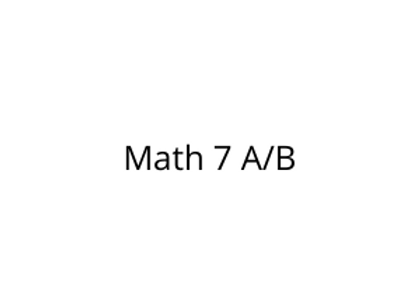# Math 7 A/B

\$395.00Price
`Mathematics is the study of the patterns around us.  Using the tools in this course, you will learn more about how to solve problems using expressions and equations.  When you understand how to work with numbers in equations, and how to manipulate equations, you can more easily solve problems you encounter in everyday life.  By the end of Semester A, you will: Identify the constant of proportionality in tables, graphs, diagrams, and descriptions of proportional relationships.  Use equations to represent proportional relationships.  Use proportional relationships to solve real-world and mathematical problems involving ratio and percent.  Apply and extend your previous understanding of operations with fractions to add, subtract, multiply, and divide rational numbers.  Convert a rational number to a decimal number using long division.  Use variables to represent quantities in a real-world or mathematical problem and write simple expressions, equations, or inequalities to solve the problem.  Use properties of operations to rewrite linear expressions in different forms.   By the end of Semester B, you will:  Solve problems that involve scale drawings of geometric figures.   Construct geometric shapes with traditional tools and with technology to satisfy given conditions.   Solve real-world and mathematical problems involving angle measure, area, surface area, and volume.   Use data from a random sample to draw inferences about a population.Compare two populations using their measures of center and measures of variability.   Understand that probability is a measure of the likelihood that a chance event will occur.   Compare expected probability to relative frequency and explain any discrepancies.   Find the probability of a compound event by identifying all the possible outcomes surrounding the event.   Design and use a simulation to generate frequencies for compound events.   `